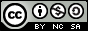- 目录 -
LeetCode 726 原子的数量(递归,map)

输入:
formula = "H2O"



输入:
formula = "Mg(OH)2"



输入:
formula = "K4(ON(SO3)2)2"



• 所有原子的第一个字母为大写，剩余字母都是小写。
• formula的长度在[1, 1000]之间。
• formula只包含字母、数字和圆括号，并且题目中给定的是合法的化学式。

## 代码

  1 2 3 4 5 6 7 8 9 10 11 12 13 14 15 16 17 18 19 20 21 22 23 24 25 26 27 28 29 30 31 32 33 34 35 36 37 38 39 40 41 42 43 44 45 46 47 48 49 50 51 52 53 54 55 56 57 58 59  class Solution { public: string countOfAtoms(string formula) { int i = 0; string ans; for (auto &kv : get_map(formula, i)) { ans += kv.first; if (kv.second > 1) ans += to_string(kv.second); } return ans; } //获结果的map map get_map(string &s, int &i) { map ans; while (i != s.length()) { if (s[i] == '(') { map tmp = get_map(s, ++i); int cnt = get_num(s, i); for (auto &kv : tmp) ans[kv.first] += kv.second * cnt; } else if (s[i] == ')') { ++i; return ans; } else { string name = get_name(s, i); int cnt = get_num(s, i); ans[name] += cnt; } } return ans; } //获取元素名字 string get_name(string &s, int &i) { string name; while (isalpha(s[i]) && (name.empty() || islower(s[i]))) name += s[i++]; return name; } //获取元素数量 int get_num(string &s, int &i) { string num; while (isdigit(s[i])) num += s[i++]; return num.empty() ? 1 : stoi(num); } };